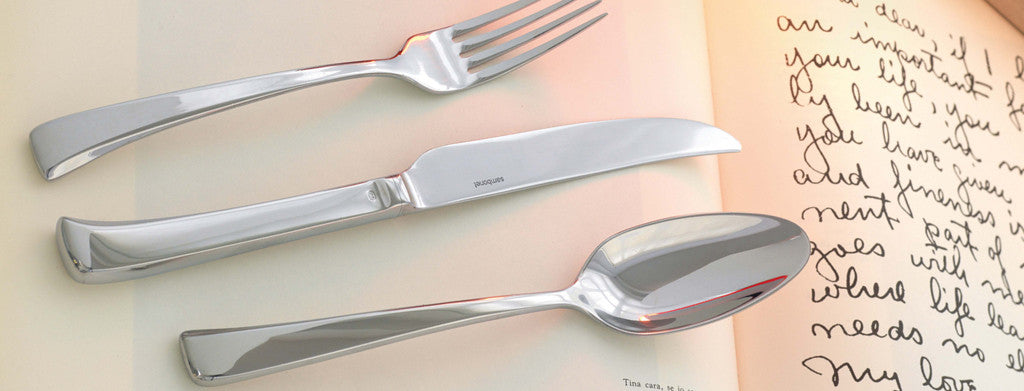Shopping Cart

### Refine

View all

#### Price# Cutlery£3.94 each (ex VAT)£6.56 each (ex VAT)£5.60 each (ex VAT)£1.73 each (ex VAT)£4.41 each (ex VAT)£3.94 each (ex VAT)£3.46 each (ex VAT)£3.46 each (ex VAT)£7.03 each (ex VAT)£7.79 each (ex VAT)£7.79 each (ex VAT)£7.79 each (ex VAT)£31.59 each (ex VAT)£0.91 each (ex VAT)£0.85 each (ex VAT)£1.14 each (ex VAT)£3.04 each (ex VAT)£3.53 each (ex VAT)£4.41 each (ex VAT)£4.54 each (ex VAT)£12.47 each (ex VAT)£7.79 each (ex VAT)£0.91 each (ex VAT)£0.91 each (ex VAT)£0.85 each (ex VAT)£0.91 each (ex VAT)£1.40 each (ex VAT)£1.25 each (ex VAT)£1.59 each (ex VAT)£1.15 each (ex VAT)£1.08 each (ex VAT)£1.02 each (ex VAT)£5.37 each (ex VAT)£4.11 each (ex VAT)£4.11 each (ex VAT)£4.74 each (ex VAT)£6.16 each (ex VAT)£3.49 each (ex VAT)£5.19 each (ex VAT)£11.55 each (ex VAT)£9.96 each (ex VAT)£9.33 each (ex VAT)£9.96 each (ex VAT)£4.64 each (ex VAT)£3.23 each (ex VAT)£4.17 each (ex VAT)£4.77 each (ex VAT)£3.36 each (ex VAT)